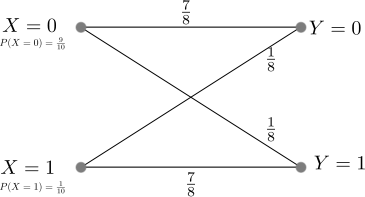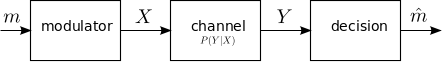- DSP log - http://www.dsplog.com -

GATE-2012 ECE Q38 (communication)

Posted By Krishna Sankar On November 3, 2012 @ 8:03 am In GATE | 13 Comments

Question 38 on Communication from GATE (Graduate Aptitude Test in Engineering) 2012 Electronics and Communication Engineering paper.

(D) 1/10Solution

The solution to the problem seem deceptively reasonably simple. Quoting from the Wiki entry on Binary Symmetric Channel 

A binary symmetric channel (or BSC) is a common communications channel model used in coding theory and information theory. In this model, a transmitter wishes to send a bit (a zero or a one), and the receiver receives a bit. It is assumed that the bit is usually transmitted correctly, but that it will be “flipped” with a small probability (the “crossover probability”). This channel is used frequently in information theory because it is one of the simplest channels to analyze.”

In our example, the transition probability i.e  probability of transition (i.e. 1 becoming 0 or 0 becoming 1) for each alphabet is given as 1/8. probability of correct transmission, for each alphabet is given as 1/8 (the probability of incorrect transition is  7/8).

The transition probability diagram with the above data can be drawn as,Figure : Transition probability diagram for a binary symmetric channel

The probability of the source alphabets are,

.

The transition probability when  is :

.

Similarly the transition probability when  is :

.

The received alphabet not equal to the transmitted alphabet is,

.

Hold on, we are not done yet.

Update (9th Nov 2012):

The above gives the probability that the received symbol is not equal to the transmitted symbol, and not the probability of error for an optimum detector. Thanks to Mr. Raghava GD who rightly pointed out in the comment section that – “the calculation that you did just gives the probability of receiving the bit erroneously and it doesn’t tell anything about the probability of error for an optimum decoder.”

After digesting his comments, and referring from Section of 1.2 of Chapter 1 of Prof. John M. Cioffi’s book  ,

Consider the channel model which produces a discrete vector output for a discrete vector input. The detector chooses a message  from all possible messages transmit vectors. The channel input vector  results in a corresponding channel output vector . The decision device translates the received vector  to an estimate of the transmitted vector  and a decoder converts  to the estimate of the message vector .Figure : Vector channel model (Reference : Figure 1.13 in Chapter 1 of Prof. John M. Cioffi’s book )

The probability of error is defined as,

.

Correspondingly, the probability of being correct is,

.

The optimum detector chooses  to minimize the probability of error  or equivalently maximize .

The probability of making the correct decision , given the received vector is

.

The above quantity known as the posteriori probability , and an optimum decision device will try to maximize the above quantity. The Maximum a Posteriori Detector (MAP), is defined as the detector that chooses the index  to maximize the a posterior probability  given the received vector .

Using Bayes therorem , the posterior probability can be written in terms of prior probability  and the channel transition probability ,

.

Note :

The term  is a constant and can be ignored when trying to maximize the posterior probability .

The MAP detection rule is,

.

Note :

This is discussed briefly in Chapter 5.1.3 The Optimum Detector in Digital Communications, Fourth edition John G. Proakis (buy from Amazon.com buy from Flipkart.com )

Applying the MAP detection rule to the problem at hand :

We have two candidates 0 and 1 for the source message i.e

a)  ,

b) modulator is a pass through i.e  and

c) received symbol  can be 0 or 1.

The goal is to make decision rule on the observed received symbol .

Applying MAP detection rule,

.

.

In both the cases i.e when received symbol , the optimum MAP detection rule suggest that the estimated message symbol is .

Intuitively, this makes sense : given that , a receiver will be better of assuming that the transmitted symbol is always 0. With this, the probability of making an error in the decision is  .

Matlab example

clear all; close all;
% number of observations
N = 10^5;
% generating x with p(x=0) = 9/10, p(x=1) = 1/10
x = (rand(1,N) > 9/10);
% generating c with p(c=0) = 1/8, p(c=1) = 7/8
c = (rand(1,N) < 7/8);
% binary symmetric channel
y = mod(x+c,2);
xhat = 0;
% counting errors
nErr    = size(find(xhat-x),2);
errProb = nErr/N

Based on the above, the right choice is (D) 1/10.

References

 GATE Examination Question Papers [Previous Years] from Indian Institute of Technology, Madras http://gate.iitm.ac.in/gateqps/2012/ec.pdf 

 Wiki entry on Binary Symmetric Channel http://en.wikipedia.org/wiki/Binary_symmetric_channel 

 Digital Communications, Fourth edition John G. Proakis (buy from Amazon.com buy from Flipkart.com )

URL to article: http://www.dsplog.com/2012/11/03/gate-2012-ece-q38-communication/

URLs in this post:

 Wiki entry on Binary Symmetric Channel: http://en.wikipedia.org/wiki/Binary_symmetric_channel

 Chapter 1 of Prof. John M. Cioffi’s book: http://www.stanford.edu/group/cioffi/book/chap1.pdf

 posteriori probability: http://en.wikipedia.org/wiki/Posterior_probability

 Bayes therorem: http://en.wikipedia.org/wiki/Bayes